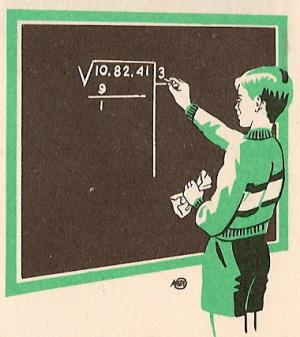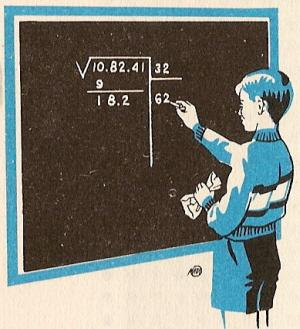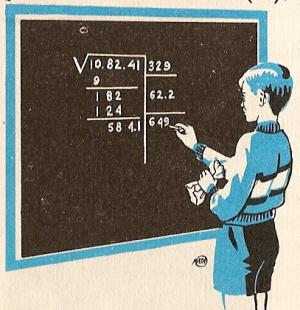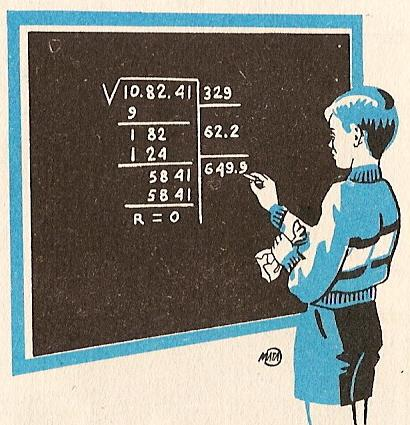1. Calculating square roots to printName: ______________________________________  Subject: _______________________ Date: _______

Write on the right side what is missing.

1. Calculating square roots

Let's find the square root of 456. On the upper picture, we see that we can make a big square (in green) with 20 points side and we have 202 = 400.
If we separate the two zeros of the right, we see that 2 =
Í4, that is, that the number of dozens of the root is equal to the whole root of the number of hundreds of the radicand.
To form another greater square, we must add 20 in a line and others in column, that is, 2 x 20 = 40, and we must also complete the upper corner. Then the number of rows that can be added will be at most the quotient among the remainder 456-400 = 56 and the duple of the root 2 x 20 = 40. This quotient is 1, and 15 points is left over.
Thus 456 = 212 + 15. The whole root is 21 and the remainder is 15.

Exercises:

 1. In  Í100, the root is... 2. In  Í400, the root is ... 3. In  Í900, the root is ... 4. In  Í1600, the root is ... 5. In  Í2500, the root is... 6. In  Í3600, the root is...

2. - Practice of the whole square root

Let's find the square root of 108 241. We will follow these 6 steps:1. We must divide 10 82 41 in groups of 2 digits, starting from the right.2. We find the square root of the number 10 which is 3, and we put it up to the right. Its square is 9 and we put it under the number 10. We subtract and we obtain 1.3. To the right of the number 1, we down the group 82. Then, we separate by a point the last number on the right (2). Under the root 3, we write the double of the root (6). The 18 is divided by 6 and we have 3. We will try to see if it the 3 is correct. We put the 3 to the right of the 6 and we have 63. We multiply it by 3 and we have 189, which is greater than 182. So the 3 is too great and we will take the 2. We put the 2 together to the 6, resulting in 624. We multiply the number 62 (.) by the last obtained number (2) and we have 124. This number is put under the number 182 and we subtract, resulting in 58. We put the 2 in the upper part next to the 3, resulting in 32.5. Then, next to the number 58 we take down the group 41 and we separate with a point the last number that is 1, resulting in 584. Under 62.2 we put a line. We calculate the double of 32 which is 64. We divide 584 by 64, resulting in 9. We put this 9 next to 64, resulting in 649. We put the number 9 with number 32, obtaining 329.6. We multiply the resulting number 649 by the number 9, resulting 649 x 9 = 5841. We put this number on the down left part, under 5841 and we found the difference. The remainder is 0. The number 329 that is in the upper right part is the exact square root of the number 108241. To verify that the operation is correct, we find 329 x 329. As the result is 108241, we conclude that the square root is well done. To do the test of the square root, we find the square root and we add the rest. The sum must be equal to the given number.

Find these square roots:

 1. The root of Í127 = 2. The remainder of  Í127 = 3. The remainder of  Í925 = 4. The root of Í1610 = 5. The root of  Í9463 = 6. The remainder of  Í9463 =

| Educational applications | Mathematics |
In Spanish  | Interactive

«Arturo Ramo GarcÝa.-Record of intellectual property of Teruel (Spain) No 141, of 29-IX-1999
Plaza Playa de Aro, 3, 1║ DO 44002-TERUEL